#Structure Factors and the Phase Problem

Intensity of reflections

In the previous lessons we have seen how we set up a diffraction experiment and what equipment we use to obtain the diffraction patterns. The question arises, how do we get from the diffraction data to a model of the actual protein structure?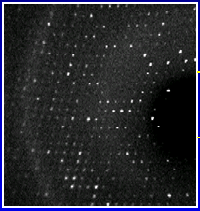A diffraction pattern as observed on a multiwire detector. The white spots are the reflections.

In a diffraction experiment we measure the intensities and the position of reflections. From the position of the reflection we can determine its index triple (h,k,l) and assign the appropriate intensity to it. This intensity is proportional to the square of the structure (factor) amplitude, |Fhkl|, and is a scalar value, i.e., a simple number. Click here to see a typical list of indexed reflections. There are usually a few ten-thousand reflections collected for each crystal.

The structure factor F(hkl)
The structure factor F(hkl) for a reflection h,k,l itself is a complex number derived quite straightforward as follows :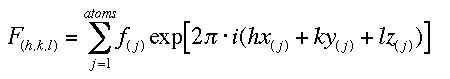(1)

This is a simple summation which extends over all atoms j, with x,y, and z their fractional coordinates. The f (j) is the scattering factor of atom j and depends on the kind of atom and the diffraction angle of the corresponding reflection (h,k,l). For h,k,l =0, f equals the atom's number of electrons. We immediately see that the dimension of F(hkl) then must be electrons. The exponent is complex (notice the i in there) with x,y,z the fractional coordinates of each atom in the summation, and h, k, l the three indices of the corresponding reflection. Formula (1) shows that if we know the structure, we can easily calculate structure factors.

You may find it helpful to calculate structure factors for a few simple structures to see how different atoms and positions change the values for F and how anomalous contributions to the atomic scattering factors, as used in MAD phasing, lead to the breakdown of Friedel's law.

In crystallographic studies we are in fact dealing with the inverse problem, we know structure factors (or, to be precise, their amplitude or magnitude only from the measured intensites) and want to know the structure. How to do that?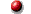Yes, I want to calculate my very own structure factors !

Fourier transform
The complex exponential function is periodic, and with the above parameters it is limited between -1,1 for its real part and -i, i for the imaginary part (its elementary strip). In such cases of periodic functions we can apply a Fourier transformation (FT) and we obtain for our formula (1) the following FT, which has now the F(hkl)s as its Fourier coefficients: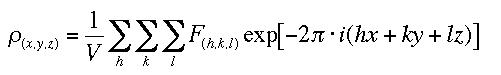(2)

We immediately see from looking at the dimensions that the result of the transformation, p(xyz), must be an electron density : F(hkl) is in units of electrons (see above) and the sum is divided by the cell volume V. We are already familiar with the rest in the summation, which now is done for each position x,y,z in the (normalized) unit cell. Note also the minus sign now preceding the exponent: In fact we are transforming an inverse space (the h,k,l's are actually derived from fractional numbers (1/h,1/k,1/l) designating where corresponding lattice planes intersect the unit cell) into a real or direct space (the electron density at a real point x,y,z in space). It is a general feature of the FT to transform from one space into its inverse space and vice versa. So our diffraction pattern (an image of the reciprocal space) it transformed back into the real space of electron density. This transformation is accurate and in principle complete. If we know the structure factors (inverse space from diffraction by electrons) we can calculate the actual real structure (the density of the electrons in real space)

.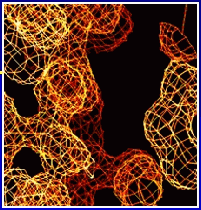This is a picture of electron density derived from actual data. The electron density is calculated for each point (x,y,z) in space and points of equal value are connected forming the characteristic wire grid presentation.

As formula (2) is essentially a summation over all structure factors, it is alse referred to as a Fourier synthesis or Fourier summation. You may want to explore and calculate electron density and Patterson maps from the structure factors you have created previously.Yes, I want to calculate electron density from my own structure factors !

Bummer
Unfortunately, a closer look at formula (2) reveals a small but bothersome detail : In order to perform the FT, we need the complex structure factors F(hkl) but we know only their magnitude |Fhkl|. In terms of physics this means that we know only the absolute value of the complex vector F(hkl) but not its phase, a(hkl). Consequently, this nuisance is called the Phase Problem. We can reformulate (2) and split it up so that the phase term a(hkl) becomes evident :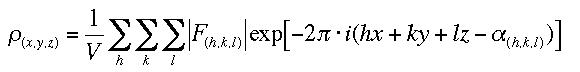(3)

In (3) we have the actually measured |Fhkl| and its corresponding unknown phase part a(hkl) separated. The question arises, how can we obtain these darn phases now to complete the transformation and get to our electron density?

We'll see that there are a number of ways to achieve this.Click on K.C.'s duck to learn more about general features of Fourier Transforms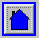Back to Introduction
This World Wide Web site conceived and maintained by Bernhard Rupp
Last revised Dezember 27, 2009 01:40